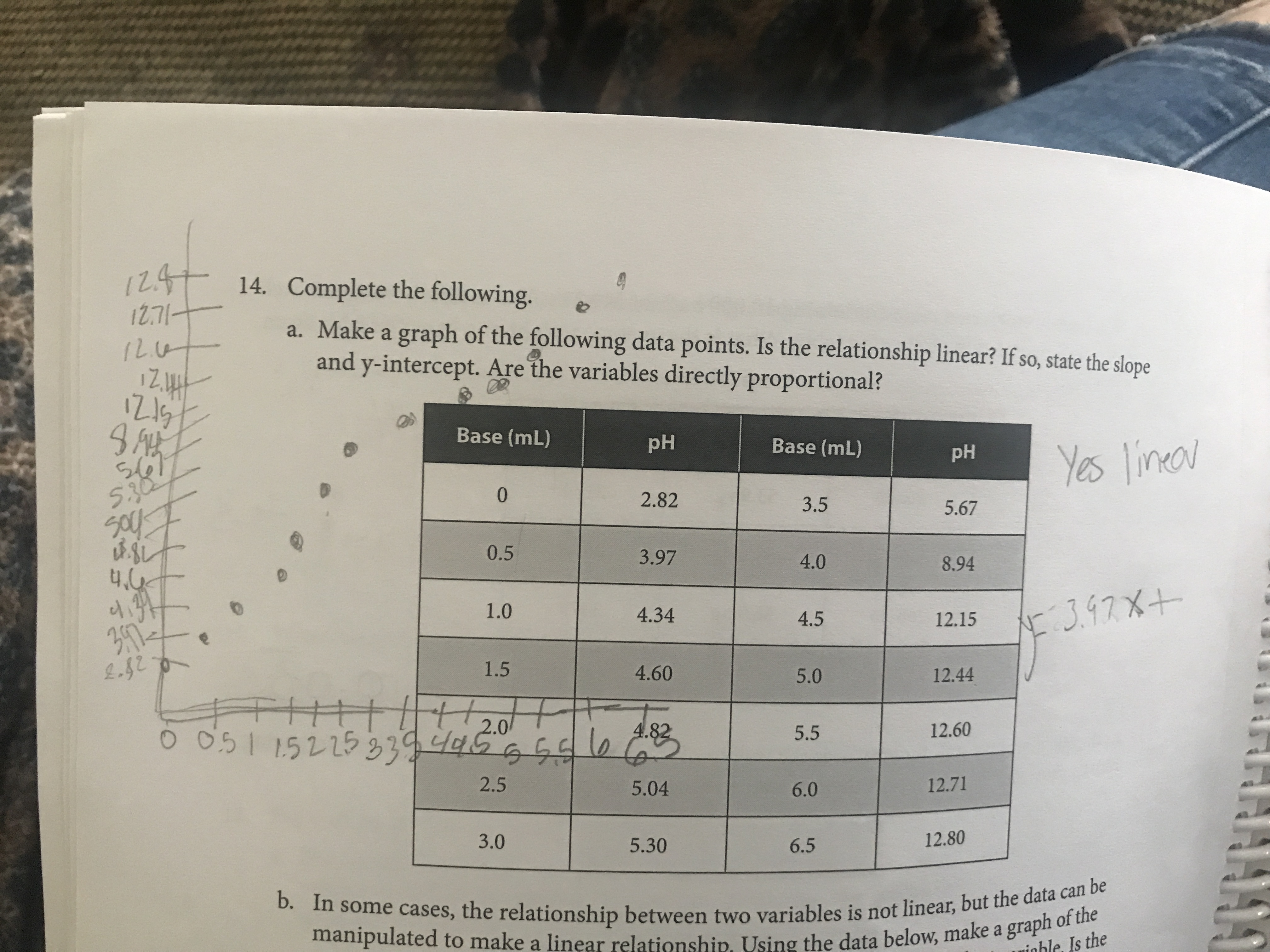# 1247 14. Complete the following.12.71-a. Make a graph of the following data points. Is the relationship linear? If so, state the slopeand y-intercept. Are the variables directly proportional?Base (mL)pHBase (mL)s675.30pHYes lineov2.823.55.670.53.974.08.941.04.344:3.97X+4.512.151.54.605.012.44TH1||||20164.825.512.602.55.046.012.713.05.306.512.80b. In some cases, the relationship between two variables is not linear, but the data can bemanipulated to make a linear relationship. Using the data below, make a graph of theIs the

Question
36 views

How do I solve this? I do know that it is linear.help_outlineImage Transcriptionclose1247 14. Complete the following. 12.71- a. Make a graph of the following data points. Is the relationship linear? If so, state the slope and y-intercept. Are the variables directly proportional? Base (mL) pH Base (mL) s67 5.30 pH Yes lineov 2.82 3.5 5.67 0.5 3.97 4.0 8.94 1.0 4.34 4:3.97X+ 4.5 12.15 1.5 4.60 5.0 12.44 TH1||||20 16 4.82 5.5 12.60 2.5 5.04 6.0 12.71 3.0 5.30 6.5 12.80 b. In some cases, the relationship between two variables is not linear, but the data can be manipulated to make a linear relationship. Using the data below, make a graph of the Is the fullscreen
check_circle

Step 1

Using the given data, graph can be drawn by plotting ...

### Want to see the full answer?

See Solution

#### Want to see this answer and more?

Solutions are written by subject experts who are available 24/7. Questions are typically answered within 1 hour.*

See Solution
*Response times may vary by subject and question.
Tagged in

### General Chemistry